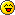Binary Converter

::  ::  ::Binary Converter

Decimal to Binary comverter
Code:
`#include <iostream>using namespace std;int main() {    int n;    while (cin >> n) {        cout << "decimal: " << n << endl;        // print binary with leading zeros        cout << "binary : ";        for (int i=31; i>=0; i--) {            int bit = ((n >> i) & 1);            cout << bit;        }        cout << endl;    }//end loop    return 0;}//end main`

Last edited by another_pd on Tue Dec 08, 2009 3:00 am; edited 1 time in totalPaul
Pickaxe

Posts : 611Re: Binary Converter

Well I hate to outdo you but I'm about to. If you are taking Cisco I wrote this program for Mr. Good and its still on all the computers in that class.

Code:
`#include "stdafx.h"#include <iostream>#include <windows.h>using namespace std;int menu();int DB();void DH();void BD();void BH();void HD();int HB();int choice;int main(){   menu();   return 0;}int menu() {   while(choice!=-1)    {   system("cls");   cout << "Welcome to Num Head. Created by Zach Bolan.\n"         "What do you want to convert?\n\n"         "1. Decimal --> Binary\n"         "2. Decimal --> Hex\n"         "3. Binary --> Decimal\n"         "4. Binary --> Hex\n"         "5. Hex --> Decimal\n"         "6. Hex --> Binary\n\n";   cout << "Choice: ";   cin>>choice;   system("cls");   switch (choice)   {   case 1:      cout<<"Converting Decimal to Binary\n\n";        DB();      break;   case 2:      cout<<"Converting Decimal to Hex\n\n";        DH();      break;    case 3:      cout<<"Converting Binary to Decimal\n\n";        BD();      break;    case 4:      cout<<"Converting Binary to Hex\n\n";        BH();      break;    case 5:      cout<<"Converting Hex to Decimal\n\n";      HD();      break;    case 6:      cout<<"Converting Hex to Binary\n\n";        HB();      break;   case 0:      cout << "Thank you for using Num Head.\n"              "Created by Zach Bolan.\n\n";      system("pause");      return 0;   default:      cerr<<"Invalid selection."<<endl;      break;   }   }return 0;}int DB (){  int num1;  char num = {00000000};  int x = 128;  int y = 0;  cout << "Number to convert: ";  cin >> num1;    if(num1>255)   {     cerr<<"\n\nYour number cannot be greater than 255.\n";     system("pause");     return -1;  }  while(y != 8)   {   if ((num1-x) >= 0)    {      num1-=x;      num[y] = '1';   }    else   {      num[y] = '0';   }   x = x / 2;   y = y + 1;  }  cout << num << endl << endl;  system("pause");  return 0;}void DH (){  long num;  cout << "Number to convert: ";  cin >> dec >> num;  cout << hex << num << endl;   system("pause");  }void BD (){   char num = {00000000}; int x = 0; int y = 128;  cout << "Number to convert: ";  cin>>num;  char num1;   int num2;  int num3 = 0;  while(x != 8)  {   num1 = num[x];   num2 = (int)num1 - 48;      if(num2 == 1)     {        num3 = num3 + y;     }     y = y / 2;     x = x + 1;  } cout << dec << num3 << endl << endl;  system("pause");}void BH (){    char num = {00000000}; int x = 0; int y = 128;  cout << "Number to convert: ";  cin>>num;  char num1;   int num2;  long num3 = 0;  while(x != 8)  {   num1 = num[x];   num2 = (int)num1 - 48;      if(num2 == 1)     {        num3 = num3 + y;     }     y = y / 2;     x = x + 1;  }  cout << hex << num3 << endl << endl;   system("pause");}void HD (){  long num;  cout << "Number to convert: ";  cin >> hex >> num;  cout << dec << num << endl;   system("pause");}int HB (){ long num1;  cout << "Number to convert: ";  cin >> dec >> hex >> num1;  char num = {00000000};  int x = 128;  int y = 0;      if(num1>255)   {     cerr<<"\n\nYour number cannot be greater than 255.\n";     system("pause");     return -1;  }  while(y != 8)   {   if ((num1-x) >= 0)    {      num1-=x;      num[y] = '1';   }    else   {      num[y] = '0';   }   x = x / 2;   y = y + 1;  }  cout << num << endl << endl;  system("pause");   return 0;}`Unchained
Mod

Posts : 448Re: Binary Converter

Notice how I said "short." I've done other stuff with base conversion and programming as well. This specific example (mine not yours) uses bit shifting and binary AND.

stdafx.h and windows.h are not necessary. I use Visual C++ as well.Paul
Pickaxe

Posts : 611Re: Binary Converter

i can count to 3Pirate King

Posts : 559Re: Binary Converter

Admin wrote:i can count to 3In binary?Paul
Pickaxe

Posts : 611Re: Binary Converter

in binary, AND english!Pirate King

Posts : 559Re: Binary Converter

stdafx.h - not required but I use precompiled headers.

windows.h actually is required, I'm using it to do a lot of the work in my conversions for me. (see the HD function)Unchained
Mod

Posts : 448Re: Binary Converter

Uno, dos, tres.
Now you can do so in Spanish too!Corey
Mod

Posts : 462Re: Binary Converter

Unchained wrote:stdafx.h - not required but I use precompiled headers.

windows.h actually is required, I'm using it to do a lot of the work in my conversions for me. (see the HD function)

What part of the code is using windows.h ?Paul
Pickaxe

Posts : 611Re: Binary ConverterLunsfordium
[Blankie]

Posts : 194Re: Binary Converter